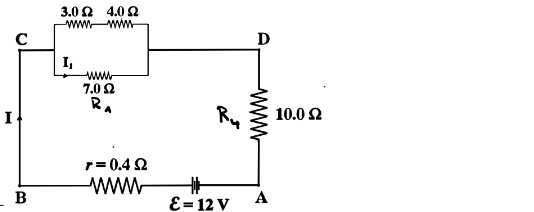# Problem: Four resistors are connected as shown in the figure to a battery with emf ε = 12 V   and internal resistance r = 0.4Ω. What is the equivalent resistance of the four resistors, i.e., not including the internal resistance of the battery?

###### Problem Details

Four resistors are connected as shown in the figure to a battery with emf ε = 12 V   and internal resistance = 0.4Ω. What is the equivalent resistance of the four resistors, i.e., not including the internal resistance of the battery?